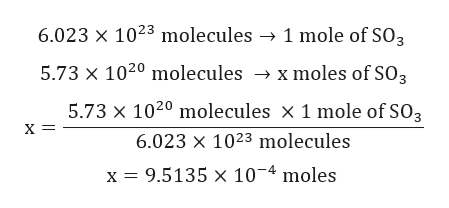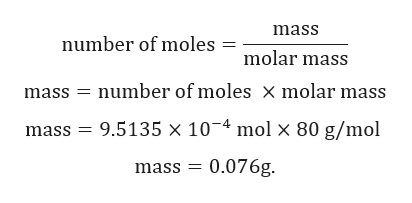Calculate the mass (in g) of each sample. 5.73x10^20 SO3 molecules

Question

Calculate the mass (in g) of each sample.

5.73x10^20 SO3 molecules

Step 1

Given:

Number of molecules = 5.73x1020 molecules.

Molar mass of SO3 = 80 g/mol.

Step 2

Calculation for number of moles of SO3:

1 mole of any substance contain 6.023x1023 molecules.help_outlineImage Transcriptionclose6.023 x 1023 molecules 1 mole of SO3 5.73 x 1020 molecules x moles of SO3 5.73 x 1020 molecules x 1 mole of SO3 х— 6.023 x 1023 molecules x = 9.5135 x 10-4 moles fullscreen
Step 3

Calculation for mass of...help_outlineImage Transcriptionclosemass number of moles molar mass mass number of moles X molar mass mass 9.5135 x 104 mol x 80 g/mol mass 0.076g. fullscreen

Want to see the full answer?

See Solution

Want to see this answer and more?

Our solutions are written by experts, many with advanced degrees, and available 24/7

See Solution
Tagged in

General Chemistry